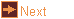# iso_delta - isohedral deltahedra

 Usage    |    Examples    |    Notes

## Usage

```

Usage: iso_delta [options] polyhedron

Make Isohedral Deltahedra in OFF format. The polyhedron may be specified
by its list number, or the (start of the) name of the polyhedron.
Additional examples can be generated using -d or -c parameters.
Based on a paper by G. C. Shephard
Periodica Mathematica Hungarica, Volume 39, Numbers 1-3, 2000 , pp. 83-106(24).
with enhancements by Jim McNeill (http://www.orchidpalms.com/polyhedra)

Options
-h,--help this help message (run 'off_util -H help' for general help)
--version version information
-l        display the list of Isohedral Deltahedra 1 thru 44
-t        generate triangle only (Isohedral Deltahedra 1 to 44 and option -d)
-v        verbose output (Isohedral Deltahedra 1 thru 44 and option -d)
-o <file> write output to file (default: write to standard output)

Isohedral Deltahedra Options
-a <ang>  angle (-c c,d,g,h,i,j,k)
-n <n/d>  n/d (d is optional) (-c a,c,g,h,k,l and -d)
note: for option -d and compound cases c, k, l:
n and d must be such that (6/5 <= n/d <= 6)
-k <int>  in special cases, for number of constituents (-c a,c,g,h,k,l)
-s <int>  in special cases, for subtypes (default: 1) (-c b,e,f,m,o)

Isohedral Deltahedra Special Cases
-d        dipyramid of n/d using -n n/d (infinite set) where 6/5 <= n/d <= 6
-c <type> compound cases (a thru f from Shephard's paper)
a - tetrahedron repeated k times, evenly spaced
when k=1 tetrahedron, when k=2 Stella Octangula
Uniform Compound Set UC23 when n/d is 2/1
b - 5 or 10 tetrahedra
s=1 icosahedral, s=2 with horizontal reflection
Uniform Compounds UC05 and UC06
c - 2 dipyramids of n/d using -a angle (default: calculated)
relaxed dual of Uniform Compound Set UC20 and k=1
d - 6 octahedra using -a angle (default: 22.5)
At 45.0 degrees is 3 Octahedra
dual of Uniform Compound Set UC07
e - 4 or 8 triangular dipyramids
s=1 octahedral, s=2 with horizontal reflection
relaxed duals of Uniform Compounds UC30 & UC31
f - 6 or 12 5/1 pentagonal or 5/2 star dipyramids
5/1: s=1 icosahedral, s=2 with horizontal reflection
relaxed duals of Uniform Compounds UC34 & UC35
5/2: s=3 icosahedral, s=4 with horizontal reflection
relaxed duals of Uniform Compounds UC36 & UC37
g - 2 tetrahedra using -a angle (default: 45.0)
At 45.0 degrees is Uniform Compound UC04
Uniform Compound Set UC23 when n/d is 2/1 and k=1
h - 2 tetrahedra repeated k times, evenly spaced
using -a angle (default: 1.0)
Uniform Compound Set UC23 when n/d is 2/1 for any k
i - 6 tetrahedra using -a angle (default: 45.0)
Uniform Compound UC01. At 45.0 degrees is UC03
j - 12 tetrahedra using -a angle (default: 30.0)
Uniform Compound UC02. At 45.0 degrees is UC03
k - 2 dipyramids of n/d repeated k times, evenly spaced
using -a angle (default: 1.0)
relaxed dual of Uniform Compound Set UC20
l - k dipyramids of n/d using -n n/d, evenly spaced
relaxed dual of Uniform Compound Set UC21
m - 10 or 20 triangular dipyramids
s=1 icosahedral, s=2 with horizontal reflection
relaxed duals of Uniform Compounds UC32 & UC33
n - 6 10/3 star dipyramids
relaxed dual of Uniform Compounds UC41
o - 5 or 10 Augmented Tetrahedra T2(1)
s=1 icosahedral, s=2 with horizontal reflection
relaxed duals of Uniform Compounds UC55 & UC56
p - 5 Augmented Octahedra O6(1)
relaxed dual of Uniform Compounds UC57
q - 5 Excavated Octahedra O6(2)
relaxed dual of Uniform Compounds UC58

Coloring Options (run 'off_util -H color' for help on color formats)
-f <mthd> mthd is face coloring method using color in map (default: c)
keyword: none - sets no color
c - unique coloring for each compound constituent
s - symmetric coloring (should always be one color)
-T <tran> face transparency. valid range from 0 (invisible) to 255 (opaque)
-m <maps> color maps for all elements to be tried in turn (default: compound)

```

## Examples

View polyhedron number 9 in the list
```iso_delta 9 | antiview
```

View 2 pairs of star-pentagon dipyramids at an angle of 3 degrees
```iso_delta -c k -n 5/2 -k 2 -a 3 | antiview
```

## Notes

iso_delta was written by Roger Kaufman.

It is based on a paper by G. C. Shephard Periodica Mathematica Hungarica, Volume 39, Numbers 1-3, 2000 , pp. 83-106(24) with enhancements by Jim McNeill and Adrian Rossiter.